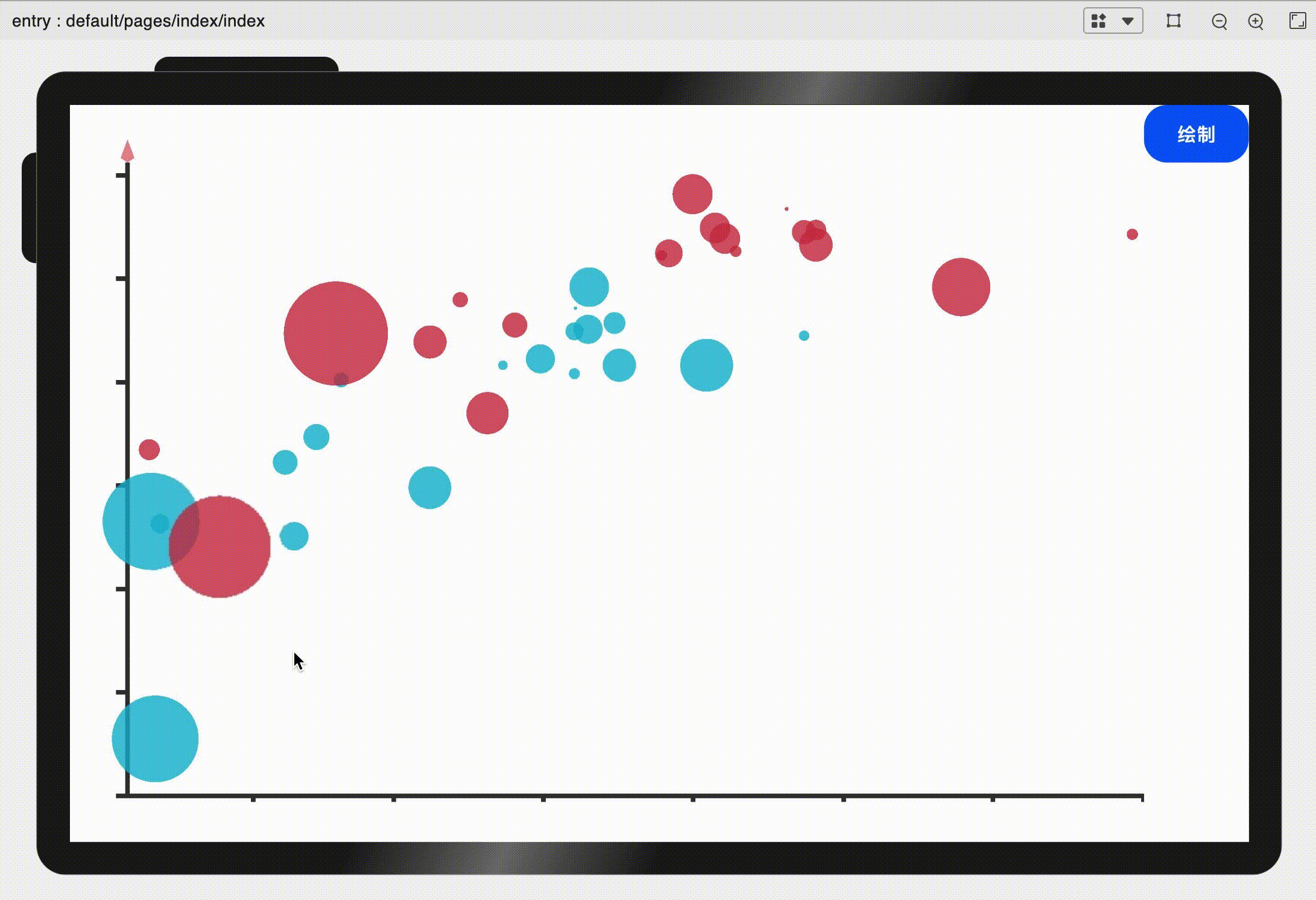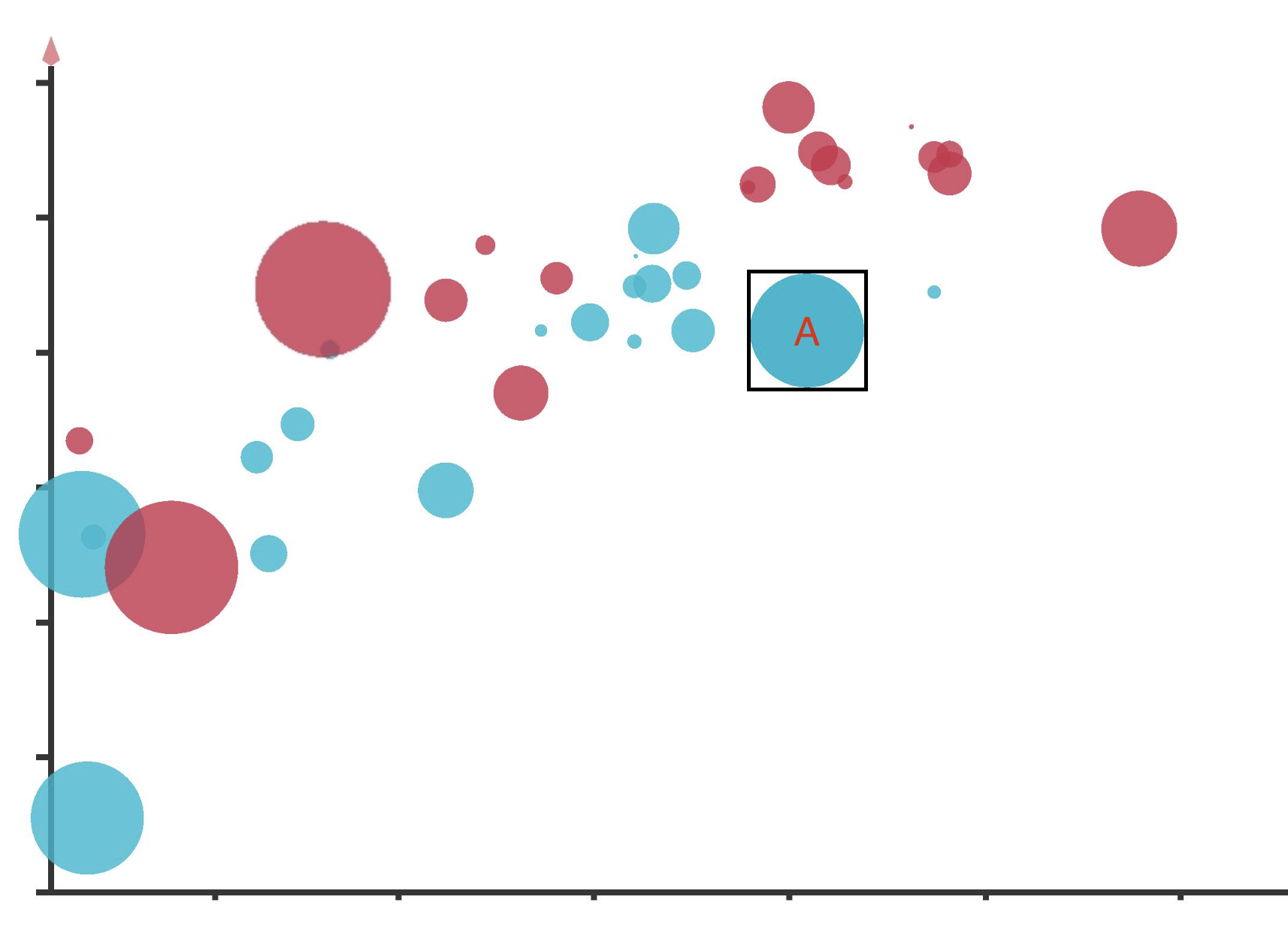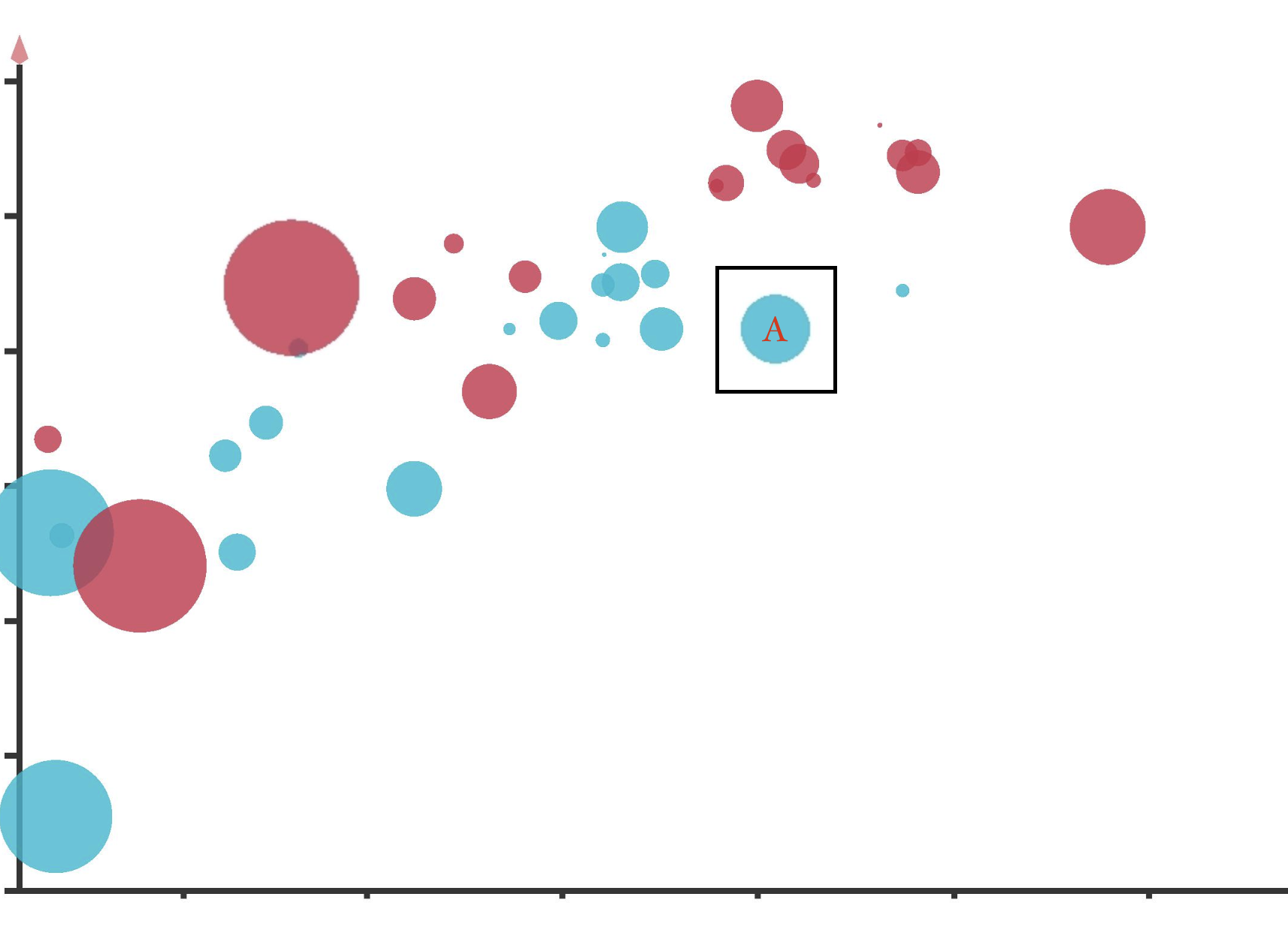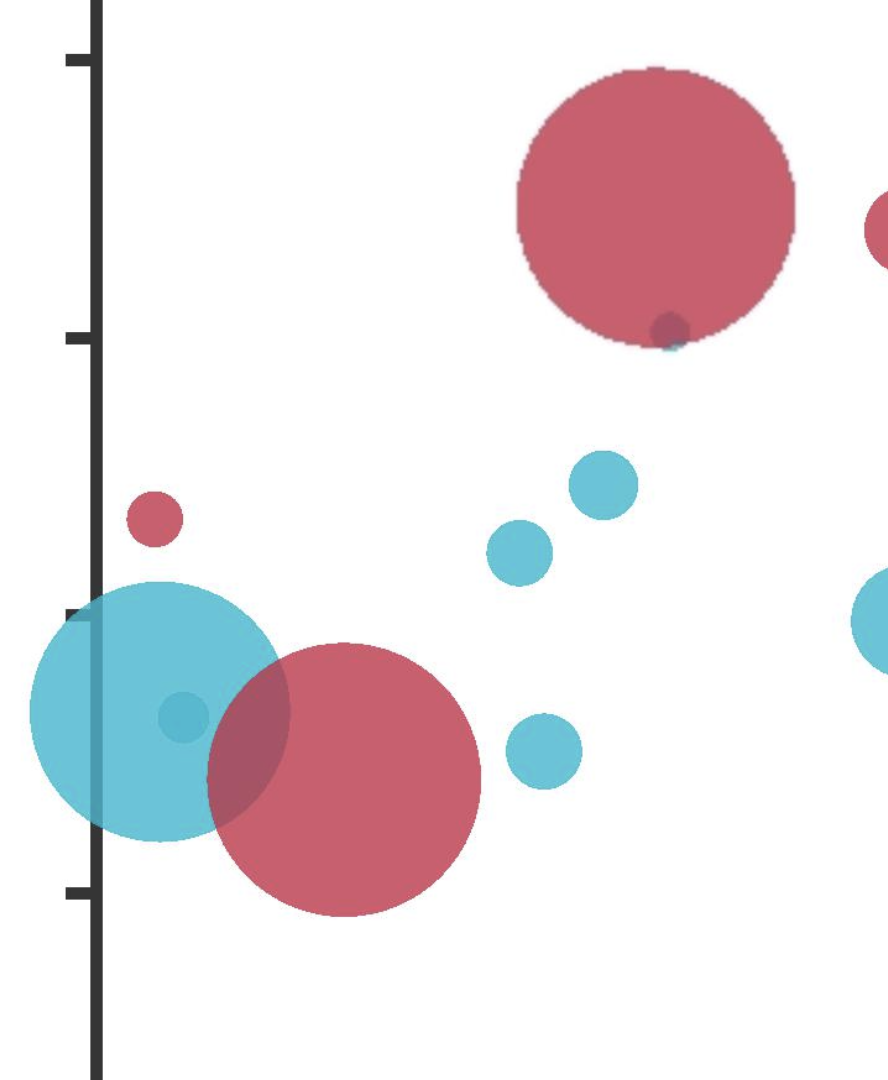# OpenHarmony——散点图 原创精华

3收藏

## 数据点的绘制

``````drawData() {
const el = this.\$element('the-canvas');
const context = el.getContext('2d');
let data = this.option.data; //获取数据集
let xLength = (this.option.chartZone - this.option.chartZone);
let yLength = (this.option.chartZone - this.option.chartZone);
let gap = xLength / this.option.xAxisLable.length;

//遍历两组数据年份
for (let i = 0; i < data.length; i++) {
let x, y, r, c;
context.fillStyle = this.option.colorPool[i]; //从颜色池中选取颜色
context.globalAlpha = 0.8; //为避免点覆盖，采取半透明绘制
//遍历各个数据点
for (let j = 0; j < data[i].length; j++) {
//计算坐标 由于举例的数据太大，我做了微调的处理，但是这违背了封装性且为魔鬼数字，不推荐
x = this.option.chartZone + xLength * data[i][j] / 70000;
y = this.option.chartZone - yLength * (data[i][j] - 55) / (85 - 55);

//散点图半径算法
//直接数值
r = data[i][j] * 5 / 100000000;
//求对数
r = Math.log(data[i][j]);
//开根号
r = Math.pow(data[i][j], 0.4) / 100;

let singleData = {
position: [x, y], radius: r, color: this.option.colorPool[i]
}
//将所有的数据点保存
this.allData.push(singleData)
//绘制散点
context.beginPath();
context.arc(x, y, r, 0, 2 * Math.PI, false);
context.fill();
context.closePath();
}

}
context.restore()
},
``````

## 实现交互1. 找到鼠标点击位置的数据点
2. 将该数据点放大
3. 点击空白处后将数据点缩小回原大小

### 将数据点缩小

1. 我们可以通过画一个和原来半径相同，圆心坐标相同的圆来实现吗？

1. 我们可以通过把多余的那部分截掉来实现吗？

`````` draw() {
this.drawBackground()
this.drawAxis()
this.drawYLables()
this.drawXLables()
this.drawData()
this.drawArrow()
this.drawArrowY()
//将canvas转成webp格式的图片
const el = this.\$element('the-canvas');
this.dataURL = el.toDataURL("image/webp", 1)
console.log("dataurl:" + this.dataURL)
this.canvas = new Image()
this.canvas.src = this.dataURL
}
````````````hover(e) {
const el = this.\$element('the-canvas');
const context = el.getContext('2d');
//获取点击的坐标
this.touchX = e.touches.globalX
this.touchY = e.touches.globalY
for (let i = 0; i < this.allData.length; i++) {
//遍历所有的数据点，判断点击的位置是否在某个数据点上
if (Math.pow((this.touchX - this.allData[i].position), 2) + Math.pow((this.touchY - this.allData[i].position), 2) <= Math.pow((this.allData[i].radius), 2)) {
context.fillStyle = this.allData[i].color;
context.globalAlpha = 0.3;
this.hoverData = {
x: this.allData[i].position,
y: this.allData[i].position,
color: this.allData[i].color
}
let step = 0.05
//如果点击的位置在数据点上，则将该数据点放大
for (let j = 0; j < 300; j++) {
//用setTimeout实现动画效果
setTimeout(() => {
context.beginPath();
//画圆
context.arc(this.allData[i].position, this.allData[i].position, this.allData[i].radius + j * step, 0, 2 * Math.PI, false);
context.fill();
context.restore()
context.closePath();
}, 300)
}
} else {
//如果不在数据点上，且没有点击过数据点，则不操作
context.globalAlpha = 1
console.log("this.hoverData:" + JSON.stringify(this.hoverData))
let x = this.hoverData.x
let y = this.hoverData.y
let r = this.hoverData.r
console.log("此时的x为：" + x)
//将图片上的部分裁剪下来贴在cavnas规定的位置上
context.drawImage(this.canvas, x - r - 15, y - r - 15, 2 * (r + 15), 2 * (r + 15), x - r - 15, y - r - 15, 2 * (r + 15), 2 * (r + 15))
context.save()
context.beginPath()
//最后画一个圆形覆盖住贴过来的图片上的圆
context.arc(x, y, r - 15, 0, 2 * Math.PI, false)
context.closePath()
context.fillStyle = "black"
context.globalAlpha = 0
context.fill()
context.restore()
}
}

},
``````## 更多原创内容请关注：深开鸿技术团队

3

1条回复

/

2022-4-8 10:18:38热门推荐
社区精华内容